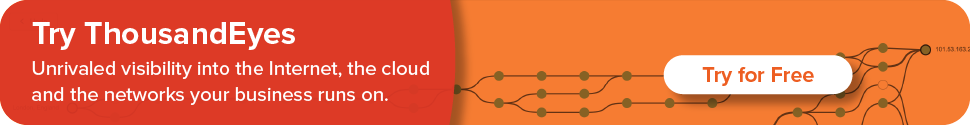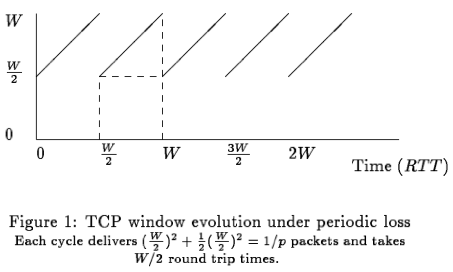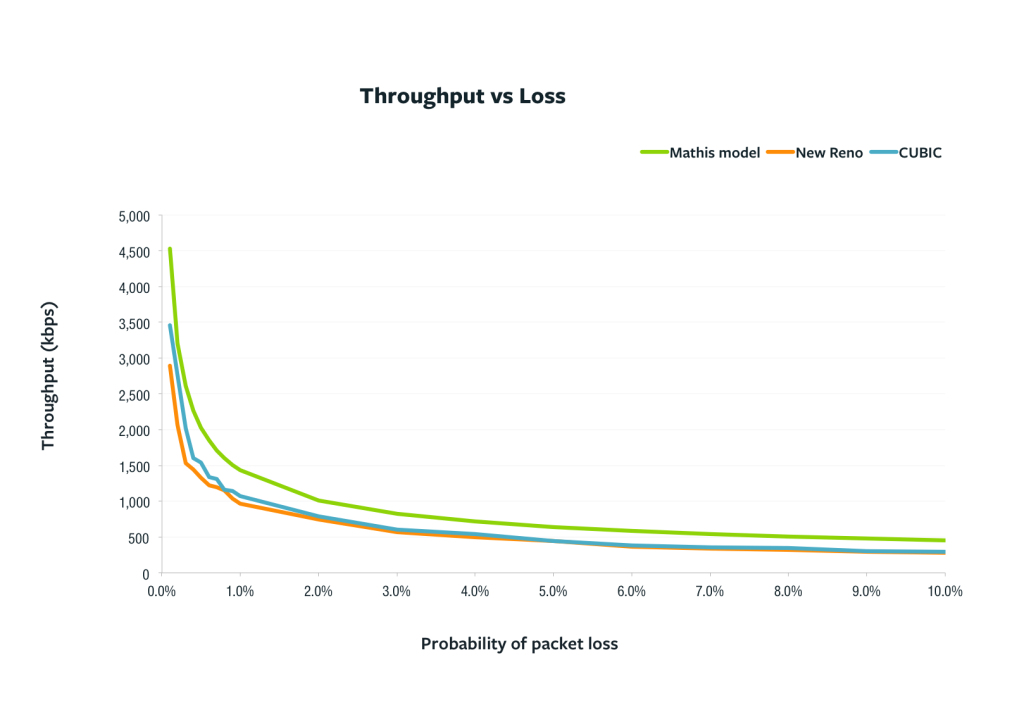Cisco Blog
Get Unique IT Insights That Help You Make The Right Decisions Fast
Product
Solutions + Industries
Learn
Free Trial
Language

# Validating A Very Simple Model for TCP Throughput

### Summary

The Mathis model is particularly appropriate to estimate the TCP throughput in network paths with regular packet loss.

Transmission Control Protocol (TCP) is the dominant underlying transport protocol in use on the Internet today. TCP is a complex protocol and predicting its throughput behavior can be hard, especially when dealing with lossy environments such as wireless networks. In this article, we look at one such model and show that it can help to predict TCP throughput behavior in the high-loss rate situations typical of wireless networks.## A Simple Model for TCP Throughput

TCP throughput, which is the rate that data is successfully delivered over a TCP connection, is an important metric to measure the quality of a network connection. TCP throughput is bounded by two mechanisms, flow control, where receiving hosts can limit the rate of incoming data to what they are able to process, and congestion control, where transmitting hosts limit their outgoing data rate to moderate their negative impact on the network. Generally speaking, TCP flow control limits throughput to a value approximating:

$Throughput=\frac{WindowSize}{RTT}$

Where WindowSize is the amount of data the receiving host is prepared to accept without explicit acknowledgment and RTT is the round-trip-time for the end-to-end network path. However, the processing capacity of internet hosts improves rapidly year on year due to the increasing availability of successively larger multicore CPUs, while the capacity of network links has historically increased at a slower rate and the latency of end-to-end network paths will always be bounded by the propagation speed of light. These historical trends have led to an increasing divergence between relative compute capacity and network performance, meaning TCP’s flow control mechanisms, which balance network performance against host processing capacity, are increasingly not the limiting factor in TCP throughput in end-to-end internet connections.

In 1997, Matthew Mathis et al., came up with a much-referenced model for the TCP throughput as bounded by its congestion control algorithms, which takes into account some link characteristics such as Maximum Segment Size (MSS), Round-Trip Time (RTT) and, most importantly, packet loss probability. Their derivation is taken from the analysis of how TCP behaves under loss during congestion avoidance phase. A TCP sender usually sends a batch of packets before receiving any acknowledgment from the receiver. If the sender sends too many packets too fast they are likely to be dropped because of congestion in intermediate queues, or will cause detrimental effects to other traffic flows by increasing congestion themselves.

To minimize this, TCP has a rate control mechanism that slows down the sender’s transmission rate if it believes devices in the path are not able to cope with the offered rate. A congestion window (cwnd) allows the sender to have at most cwnd unacknowledged bytes at any given time, effectively controlling the rate at which data is sent. There are different algorithms to control the behavior of congestion window, of which the most well known are New Reno and CUBIC. Both attempt to conservatively maximize collective network throughput while minimizing network congestion. In New Reno, a steady-state TCP connection (ie. one which has left the initial slow-start phase that attempts to rapidly estimate network capacity), cwnd grows linearly one packet per RTT and when there’s a loss event (ie. three duplicate ACKs) cwnd is set to half its value, as indicated in the figure below, extracted from the original Mathis paper.Assuming the path has a packet loss of probability p, the sender will be able to send an average of 1/p packets before a packet loss. Under this model cwnd never exceeds a maximum W because at approximately 1/p packets a new packet loss makes cwnd drop to half of its value again. Hence, the total data delivered at every cycle of 1/p packets, is given by $1/p=(\frac{w}{2})^2+\frac{1}{2}(\frac{w}{2})^2=\frac{3}{8}W^2$ (i.e., the area under sawtooth). Therefore, $MSS\times\frac{3}{8}W^2$ bytes are sent at every cycle of $RTT\times \frac{W}{2}$, which solving $W=\sqrt{\frac{8}{3p}}$ we arrive at the following throughput:

$T=\frac{MSS\times \frac{3}{8}W^2}{RTT\times \frac{W}{2}} = \frac{MSS/p}{RTT\sqrt{\frac{2}{3p}}}$

Collecting all the constant terms in $C=\sqrt{\frac{3}{2}}$, we get:

$T=\frac{MSS\times C}{RTT \times\sqrt{p}}$

This constant C lumps together several terms that are typically constant for a given combination of TCP implementation, ACK strategy (delayed vs non-delayed), and loss mechanism.

## A Simple Validation

The following is an experiment between two hosts to validate the Mathis TCP throughput model. Host A sends traffic for 30 seconds to host B on a link configured to emulate different packet loss probabilities.

We used netem utility to set up the network interface with a constant latency of 100 ms (50 ms in each host) and a loss probability from 1% to 10% (on all packets leaving host A).

host_B# tc qdisc add dev eth0 root netem latency 50ms
host_A# tc qdisc add dev eth0 root netem latency 50ms loss 2%

Iperf was used to measure the throughput from host A to host B by transmitting data during 30 seconds (-t) and using different congestion window algorithms: CUBIC (default in the Linux kernel from v2.6.19 to v3.2) and New Reno (the more traditional scheme).

host_B# iperf -s
host_A# iperf -c host_B -t 30 -Z cubicFigure 2: Comparison of estimated throughput with loss in different models.

The graph above compares the estimated throughput (from the Mathis model) with the actual measured values under different packet loss scenarios. To filter out any outliers each data point is the median of five repeated experiments.

The graph shows that the model approximates the curve of the validation measurements and demonstrates that TCP throughput is very sensitive to loss. We can see that, in practice, TCP congestion control algorithms will be more conservative than might be predicted by the model, leading to measured throughput around a third less than estimated. Loss is determinant because loss events regularly cap cwnd, the sender will never send more than W bytes at every RTT, effectively limiting its maximum throughput. So, for an average of 1% of packet loss, the TCP throughput will be restricted to 1,400 kbps (because at approximately every 100 packets a packet is lost and cwnd drops to half).

The shape of the curves also reveal that small loss rates dramatically affect TCP throughput, even at low values. For instance, the throughput drops to half from 0.1% to 0.4% of packet loss.

The Mathis model is particularly appropriate to estimate the TCP throughput in network paths with regular packet loss, which happens in a steady state when there’s a constant rate of inelastic cross traffic in the path, as might be seen in heavily loaded data center environments. If no loss event is observed, cwnd steadily increases until a corrupted packet is detected (e.g., wireless environment) or the receiver advertises an insufficient receive buffer. The model also works in lossy wireless links with a uniform loss probability.

Loss is not a constant characteristic of a network path. Instead, it varies according to different network conditions, such as the physical capacity of the links (and in particular of the bottleneck link) or network congestion, which delimits the available bandwidth. We talk more about network capacity and available bandwidth in a follow up post.